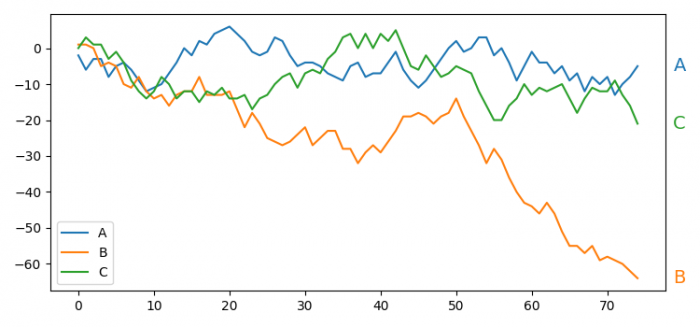# How to annotate the end of lines using Python and Matplotlib?

To annotate the end of lines using Python and Matplotlib, we can take the following steps −

• Set the figure size and adjust the padding between and around the subplots.
• Initalize a variable, rows, to get the number of rows data.
• Get a Pandas dataframe in a rectangular tabular data.
• Calculate the cumsum (cumulative sum) of the dataframe.
• Plot the dataframe using plot() method.
• Iterate line and name to annotate the end of lines.
• Use annotate() method with column's name, xy co-ordinates, color of the lines, sizes, etc.
• Place a legend on the figure.
• To display the figure, use show() method.

## Example

import pandas as pd
import numpy as np
import matplotlib.pyplot as plt

plt.rcParams["figure.figsize"] = [7.50, 3.50]
plt.rcParams["figure.autolayout"] = True

rows = 75

df = pd.DataFrame(np.random.randint(-5, 5, size=(rows, 3)),
columns=['A', 'B', 'C'])
df = df.cumsum()
ax = df.plot()

for line, name in zip(ax.lines, df.columns):
y = line.get_ydata()[-1]
ax.annotate(name, xy=(1, y), xytext=(6, 0),
color=line.get_color(), xycoords=ax.get_yaxis_transform(),
textcoords="offset points", size=14, va="center")

plt.legend(loc='lower left')
plt.show()

## Output